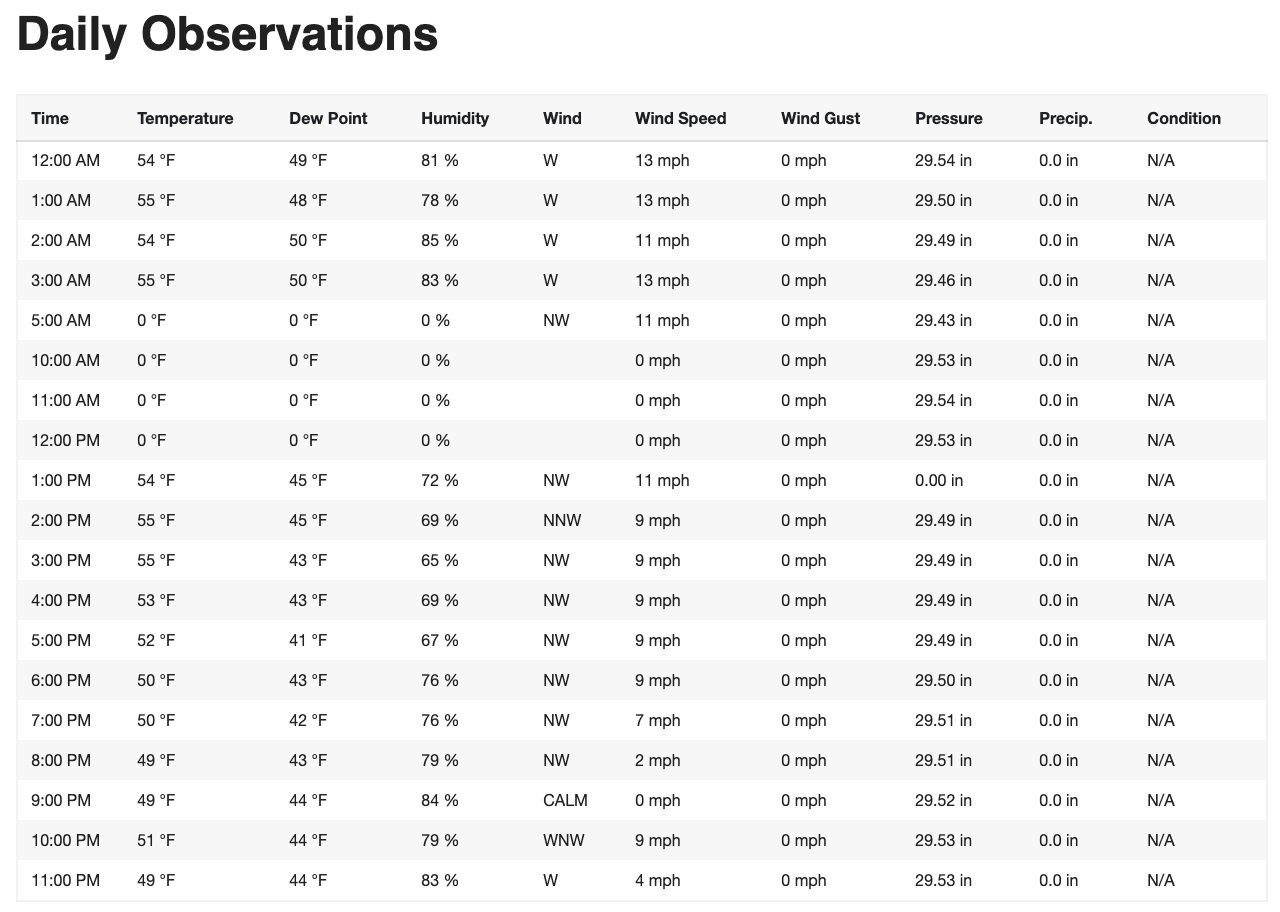# Content

### Web Scraping Wunderground Weather History With Python - Daily

This article is also related to scraping Wunderground data, although in this case I'm showing the code for scraping daily instead of monthly historical data. In this case you can obtain hourly data per day. The code works similarly to the one I have shown on my previous article.

Firstly we import the necessary libraries:

```                ```
from bs4 import BeautifulSoup as BS
from selenium import webdriver
from functools import reduce
import pandas as pd
import time
from selenium.webdriver.common.by import By
from selenium.webdriver.support.ui import WebDriverWait
from selenium.webdriver.support import expected_conditions as EC
```
```

Then the function for rendering the page is pretty much the same, although I have now added an extra functionality which allows you to choose the measurement system you want to extract the data in. For Celsius you have to pass "C" and for Fahrenheit "F". Keep in mind that extracting data in Fahrenheit is much faster. This would matter only if you are trying to get a big amount of data. Also don't forget to change the path to the chromedriver. In case you need an explanation about the chromedriver, please read the first Wunderground Scraper article.

```                ```
def render_page(url,type):
driver = webdriver.Chrome('/path_to/chromedriver')
driver.get(url)
time.sleep(3)
if type =="C":
element = WebDriverWait(driver, 10).until(
EC.presence_of_element_located((By.ID, 'wuSettings'))
)
element.click()
element = WebDriverWait(driver, 10).until(
EC.presence_of_element_located((By.XPATH, '//*[@id="wuSettings-quick"]/div/a')))
element.click()
time.sleep(3)
r = driver.page_source
driver.quit()
if type=="F":
r = driver.page_source
driver.quit()
return r
```
```

Next we run the scraper which extracts and converts all the information from the table in the bottom of the page as pictured below.```                ```
def hourly_scraper(page,dates,type):
output = pd.DataFrame()

for d in dates:
url = str(str(page) + str(d))

r = render_page(url,type)

soup = BS(r, "html.parser",)
container = soup.find('lib-city-history-observation')
check = container.find('tbody')

data = []
data_hour = []
for i in check.find_all('span', class_='ng-star-inserted'):
trial = i.get_text()
data_hour.append(trial)

for i in check.find_all('span', class_='wu-value wu-value-to'):
trial = i.get_text()
data.append(trial)

numbers = pd.DataFrame([data[i:i+7] for i in range(0, len(data), 7)],columns=["Temperature","Dew Point","Humidity","Wind Speed","Wind Gust","Pressure","Precipitation"])
hour = pd.DataFrame(data_hour[0::17],columns=["Time"])
wind = pd.DataFrame(data_hour[7::17],columns=["Wind"])
condition = pd.DataFrame(data_hour[16::17],columns=["Condition"])

dfs = [hour,numbers,wind,condition]

df_final = reduce(lambda left, right: pd.merge(left, right, left_index=True, right_index=True), dfs)
df_final['Date'] = str(d)

output = output.append(df_final)
print(str(str(d) + ' finished!'))

return output

page = "https://www.wunderground.com/history/daily/pt/lisbon/LPPT/date/"
dates = ["2020-12-27"]

hourly = hourly_scraper(page,dates,"C")
```
```